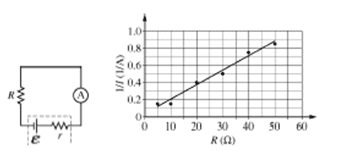# Internal Resistance of a Battery homework

• iamnotlegend
In summary, the students attempted to find the internal resistance of the battery by graphing the inverse of current as a function of resistance and using the loop rule. They found that the internal resistance would be the y-intercept on the graph.

## Homework Statement

Students are given some resistors, a battery with internal resistance, and an ammeter. They are asked to determine the emf e and internal resistance of the battery using just this equipment. Working with the circuit shown at right, they insert each resistor into the circuit and measure the current I in the circuit each time they insert a resistor. From their data, students generate a graph of the inverse of their current (1/I) as a function of the resistance of each resistor. Find the internal resistance of the battery.## Homework Equations

Kirchoff’s 2nd rule or loop rule is based on conservation of energy. The loop rule is that the sum of changes in potential around any closed loop of a circuit must be zero. For this circuit we have three changes in potential.

We have the battery, internal resistor, and the added resistor that all results in potential changes.

We will say the battery is positive and the resistors cause a negative (drop) in potential, so the sum is:[/B]

E-IR-Ir=0
where E=electrostatic motion, I=current, R=resistance, and r=internal resistance

## The Attempt at a Solution

E=IR+Ir
E=I(R+r)
E/I=R+r
r=(E/I)-R

Looking at the graph, some people say that the internal resistance would be the x-intercept. Here 1/I=0, so current would be infinite. This is the point where there is zero resistance in the system

But if I solve this equation for inverse of current I get

Inverse of current=R/E+r/E
The resistance would be our x-values for this graph. I was thinking that the 1/E would be like the slope while r/E is the y-intercept.
I am not sure what to do. Could someone help clarify?

iamnotlegend said:
The resistance would be our x-values for this graph. I was thinking that the 1/E would be like the slope while r/E is the y-intercept.
Sounds like a good approach. What values do you get for E and r if you do it this way?

gneill said:
Sounds like a good approach. What values do you get for E and r if you do it this way?
Wait, so we could think of the internal resistance of the battery as the y-intercept?

I mean use the y-intercept to find the r value?

iamnotlegend said:
I mean use the y-intercept to find the r value?
Sure. But you might want to determine E first, since the expression for the y-intercept involves both E and r...

Well I find the slope of 1/E to be about 1/60, so E would be 60 I think?

iamnotlegend said:
Well I find the slope of 1/E to be about 1/60, so E would be 60 I think?
60 what? Units are important if you want full marks ;)

So, carry on from there and see what you get for r. Using those values for E and r can you reproduce the graph using your basic circuit laws?

Oh sorry 60 volts. I took the slope of the line and then inverted it to get the result. m=y_2-y_1/x_2-x_1=.8-.3/45-115=.5/30=1/60, 1/E=1/60, E=60 volts, so E=I(R+r), 1/I=1/E(R+r)

r=(E/I)-R
r=(60/(1/0.3))-15
r=(60*0.3)-15
r=3 ohms

Okay, looks reasonable. Now pencil in those component values in your circuit and drop in a couple of values for R to see if the current that results matches your "measured" values per the given graph. This is a way to confirm your results (it's always helpful if you can find a way to check your answer).